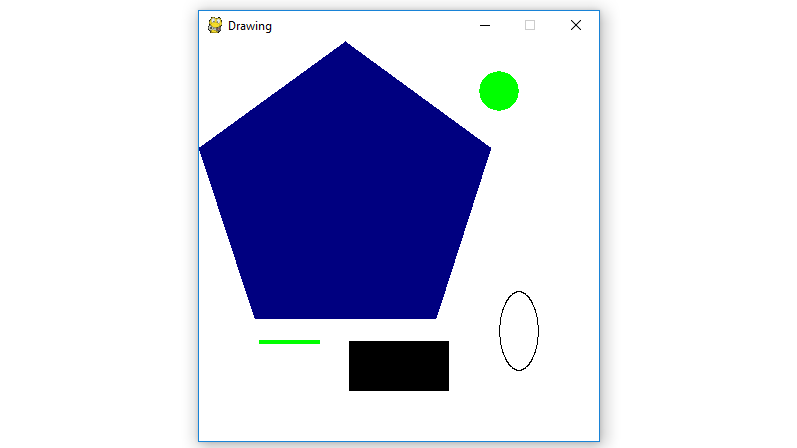Open in App
Not now

# Python | Drawing different shapes on PyGame window

• Difficulty Level : Medium
• Last Updated : 14 Jan, 2019

`Pygame` is a cross-platform set of Python modules designed for writing video games. It includes computer graphics and sound libraries designed to be used with the Python programming language. Now, it’s up to the imagination or necessity of developer, what type of game he/she wants to develop using this toolkit.

Command to install pygame :

```pip install pygame
```

There are four basic steps to displaying images on the `pygame` window :

• Create a display surface object using `display.set_mode()` method of pygame.
• Completely fill the surface object with white using `fill()` method of pygame display surface object.
• Drawing different shapes onto a surface object using `Primitive Drawing Functions` of pygame.
• Show the display surface object on the pygame window using `display.update()` method of pygame.

Below is the implementation:

 `# import pygame module in this program``import` `pygame`` ` `# activate the pygame library .``# initiate pygame and give permission``# to use pygame's functionality.``pygame.init()`` ` `# define the RGB value``# for white, green,``# blue, black, red``# colour respectively.``white ``=` `(``255``, ``255``, ``255``)``green ``=` `(``0``, ``255``, ``0``)``blue ``=` `(``0``, ``0``, ``128``)``black ``=` `(``0``, ``0``, ``0``)``red ``=` `(``255``, ``0``, ``0``)`` ` `# assigning values to X and Y variable``X ``=` `400``Y ``=` `400`` ` `# create the display surface object``# of specific dimension..e(X,Y).``display_surface ``=` `pygame.display.set_mode((X, Y ))`` ` `# set the pygame window name``pygame.display.set_caption(``'Drawing'``)`` ` `# completely fill the surface object ``# with white colour ``display_surface.fill(white)`` ` `# draw a polygon using draw.polygon()``# method of pygame.``# pygame.draw.polygon(surface, color, pointlist, thickness)``# thickness of line parameter is optional.``pygame.draw.polygon(display_surface, blue,``                    ``[(``146``, ``0``), (``291``, ``106``),``                    ``(``236``, ``277``), (``56``, ``277``), (``0``, ``106``)])``                     ` `# draw a line using draw.line()``# method of pygame.``# pygame.draw.line(surface, color,``# start point, end point, thickness) ``pygame.draw.line(display_surface, green,``                ``(``60``, ``300``), (``120``, ``300``), ``4``)`` ` `# draw a circle using draw.circle()``# method of pygame.``# pygame.draw.circle(surface, color,``# center point, radius, thickness) ``pygame.draw.circle(display_surface,``           ``green, (``300``, ``50``), ``20``, ``0``)`` ` `# draw a ellipse using draw.ellipse()``# method of pygame.``# pygame.draw.ellipse(surface, color,``# bounding rectangle, thickness) ``pygame.draw.ellipse(display_surface, black,``                    ``(``300``, ``250``, ``40``, ``80``), ``1``)`` ` `# draw a rectangle using draw.rect()``# method of pygame.``# pygame.draw.rect(surface, color,``# rectangle tuple, thickness)``# thickness of line parameter is optional.``pygame.draw.rect(display_surface, black,``                    ``(``150``, ``300``, ``100``, ``50``))`` ` `# infinite loop``while` `True` `:``     ` `    ``# iterate over the list of Event objects``    ``# that was returned by pygame.event.get() method.``    ``for` `event ``in` `pygame.event.get() :`` ` `        ``# if event object type is QUIT``        ``# then quitting the pygame``        ``# and program both.``        ``if` `event.``type` `=``=` `pygame.QUIT :`` ` `            ``# deactivates the pygame library``            ``pygame.quit()`` ` `            ``# quit the program.``            ``quit()`` ` `        ``# Draws the surface object to the screen. ``        ``pygame.display.update() `

Output :My Personal Notes arrow_drop_up